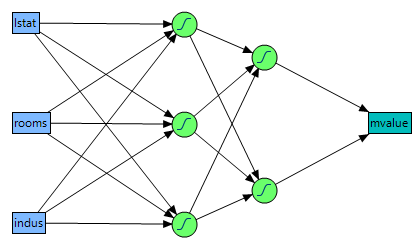Predictive and Specialized Modeling > Neural Networks > Overview of the Neural Platform
Publication date: 04/12/2021

# Overview of the Neural Platform

Think of a neural network as a function of a set of derived inputs, called hidden nodes. The hidden nodes are nonlinear functions of the original inputs. You can specify up to two layers of hidden nodes, where each layer can contain as many hidden nodes as you want.

Figure 3.2 shows a two-layer neural network with three X variables and one Y variable. In this example, the first layer has two nodes, and each node is a function of all three nodes in the second layer. The second layer has three nodes, and all nodes are a function of the three X variables. The predicted Y variable is a function of both nodes in the first layer.

Figure 3.2 Neural Network DiagramThe functions applied at the nodes of the hidden layers are called activation functions. The activation function is a transformation of a linear combination of the X variables. For more information about the activation functions, see Hidden Layer Structure.

The function applied at the response is a linear combination (for continuous responses), or a logistic transformation (for nominal or ordinal responses).

The main advantage of a neural network model is that it can efficiently model different response surfaces. Given enough hidden nodes and layers, any surface can be approximated to any accuracy. The main disadvantage of a neural network model is that the results are not easily interpretable. This is because there are intermediate layers rather than a direct path from the X variables to the Y variables, as in the case of regular regression.

Want more information? Have questions? Get answers in the JMP User Community (community.jmp.com).